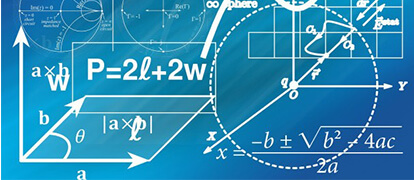# 6 Common Errors In Year 12 Maths Extension 2 Integration

You must avoid these common integration errors in your Maths Extension 2 exams.Avoid the 6 Common Errors In Year 12 Maths Extension 2 Integration! Learn the errors HSC students are making and avoid them.

## Mistake #1: Confusing Integration and Differentiation

Confusion with the difference between $$(ln \ x){^2}$$ and $$ln \ x{^2}$$ and consequently errors made in differentiation or integration of these functions.

These two functions are not equal!

### Example:

$$ln \ x{^2} = 2 \ ln \ x$$ whereas $$(ln \ x){^2} = (ln \ x) \times (ln \ x)$$

Hence, $$\frac {d}{dx} (ln \ x{^2}) = \frac{d}{dx} [2 \ ln \ x] = 2 \times \frac{1}{x}$$

$$\frac{d}{dx}(ln \ x{^2}$$ requires the use of the chain rule.

$$\frac{d}{dx} (ln \ x){^2} = 2 \ ln \ x \times \frac{1}{x}$$

## Mistake #2: Wrong Function

Incorrect choice of the function for $$\frac{dv}{dx}$$ and for $$u$$.

### Example:

LIATE (Logarithmic, Inverse, Trigonometric, Exponential) is an order that you could use to determine which function is represented by $$u$$ when the integrand is a product of any two of these functions.

$$\int x{^2} dx$$ use $$u= ln \ x$$$$\frac{dv}{dx}=x{^2}$$ (since $$ln \ x$$ and for $$x{^2}$$ is algebraic)

## Mistake #3: Differentiate of Integrate?

Other common errors are from $$\frac{dv}{dx}$$ you need to integrate and not differentiate to get $$v$$ and you need to differentiate $$u$$ to ger $$\frac{du}{dx}$$.

### Example:

$$\frac{dv}{dx}=x{^2}$$ therefore $$v=2x$$ (you have differentiated, not integrated). It should be $$\frac{dv}{dx}=x{^2}$$ then $$v=\frac{x{^3}}{3}$$

In your solutions for the integral of products involving the use of integration by parts:

• Always define the functions representing $$u$$ and $$/frac{dv}{dx}$$ and find $$/frac{du}{dx}$$ by differentiating and $$v$$ by integrating $$\frac{dv}{dx}$$
• Write out the formula for the method of integration by parts and substitute into the formula.

## Mistake #4: Incorrect Choice of Methods Of Integration

When integrating products, first check that one of the functions cannot be expressed as a derivative of the other function. If it can, then integration by parts is not the correct technique. An appropriate substitution may lead to a standard integral.

### Example:

$$\int e{^{\cos x}}\sin x \ dx$$ By using the substitution $$u= \cos x$$ will lead to a standard integral.

## Mistake #5: Not Changing The Variable Back To the Original

When using a substition in indefinite integrals, the final answer is often not rewritten in terms of the original variable.

### Example:

$$\int\frac{dx}{1+\cos x}$$ using the substition $$t=\tan\frac{x}{2}$$. The substitution changes the variable back to $$x$$ using $$t=\tan\frac{x}{2}$$

## Mistake #6: Not Changing The Limits

For definite integrals, one of the common errors is the failure to also change the limits. There is no need to rewrite the answer in terms of the original variable for definite integrals.

### Example:

Evaluate $$\int_1^3 \frac{dx}{x\sqrt{2x+1}}$$

Using the substitution $$u{^2}=2x+1$$, the limits will change from $$x=1$$ to $$u=\sqrt{3}$$ and $$x=3$$ to $$u=\sqrt{7}$$.

These tips were written by Matrix Education’s former Head Maths Teacher Pat Rockett.

## Do you want help avoiding these Extension 2 errors?